$$\newcommand{\id}{\mathrm{id}}$$ $$\newcommand{\Span}{\mathrm{span}}$$ $$\newcommand{\kernel}{\mathrm{null}\,}$$ $$\newcommand{\range}{\mathrm{range}\,}$$ $$\newcommand{\RealPart}{\mathrm{Re}}$$ $$\newcommand{\ImaginaryPart}{\mathrm{Im}}$$ $$\newcommand{\Argument}{\mathrm{Arg}}$$ $$\newcommand{\norm}{\| #1 \|}$$ $$\newcommand{\inner}{\langle #1, #2 \rangle}$$ $$\newcommand{\Span}{\mathrm{span}}$$

# 3.E: Polynomial and Rational Functions(Exercises)

$$\newcommand{\vecs}{\overset { \rightharpoonup} {\mathbf{#1}} }$$ $$\newcommand{\vecd}{\overset{-\!-\!\rightharpoonup}{\vphantom{a}\smash {#1}}}$$$$\newcommand{\id}{\mathrm{id}}$$ $$\newcommand{\Span}{\mathrm{span}}$$ $$\newcommand{\kernel}{\mathrm{null}\,}$$ $$\newcommand{\range}{\mathrm{range}\,}$$ $$\newcommand{\RealPart}{\mathrm{Re}}$$ $$\newcommand{\ImaginaryPart}{\mathrm{Im}}$$ $$\newcommand{\Argument}{\mathrm{Arg}}$$ $$\newcommand{\norm}{\| #1 \|}$$ $$\newcommand{\inner}{\langle #1, #2 \rangle}$$ $$\newcommand{\Span}{\mathrm{span}}$$ $$\newcommand{\id}{\mathrm{id}}$$ $$\newcommand{\Span}{\mathrm{span}}$$ $$\newcommand{\kernel}{\mathrm{null}\,}$$ $$\newcommand{\range}{\mathrm{range}\,}$$ $$\newcommand{\RealPart}{\mathrm{Re}}$$ $$\newcommand{\ImaginaryPart}{\mathrm{Im}}$$ $$\newcommand{\Argument}{\mathrm{Arg}}$$ $$\newcommand{\norm}{\| #1 \|}$$ $$\newcommand{\inner}{\langle #1, #2 \rangle}$$ $$\newcommand{\Span}{\mathrm{span}}$$

## 3.1 Complex Numbers

#### Verbal

1) Explain how to add complex numbers.

Add the real parts together and the imaginary parts together.

2) What is the basic principle in multiplication of complex numbers?

3) Give an example to show the product of two imaginary numbers is not always imaginary.

$$i$$ times $$i$$ equals $$-1$$, which is not imaginary (answers vary)

4) What is a characteristic of the plot of a real number in the complex plane?

#### Algebraic

For the exercises 5-10, evaluate the algebraic expressions.

5) If $$f(x)=x^2+x−4$$, evaluate $$f(2i)$$.

$$−8+2i$$

6) If $$f(x)=x^3−2$$, evaluate $$f(i)$$.

7) If $$f(x)=x^2+3x+5$$,evaluate $$f(2+i)$$.

$$14+7i$$

8) If $$f(x)=2x^2+x−3$$, evaluate $$f(2−3i)$$.

9) If $$f(x)=\dfrac{x+1}{2−x}$$, evaluate $$f(5i)$$.

$$−\dfrac{23}{29}+\dfrac{15}{29}i$$

10) If $$f(x)=\dfrac{1+2x}{x+3}$$, evaluate $$f(4i)$$.

#### Graphical

For the exercises 11-12, determine the number of real and nonreal solutions for each quadratic function shown.

11)$$2$$ real and $$0$$ nonreal

12)For the exercises 13-16, plot the complex numbers on the complex plane.

13) $$1−2i$$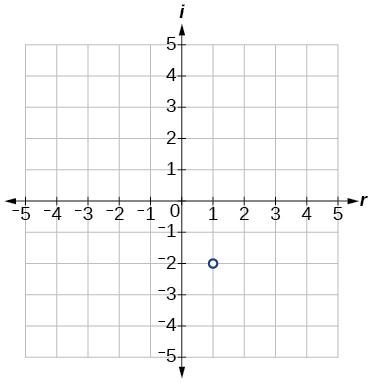14) $$−2+3i$$

15) $$i$$16) $$−3−4i$$

#### Numeric

For the exercises 17-43, perform the indicated operation and express the result as a simplified complex number.

17) $$(3+2i)+(5−3i)$$

$$8−i$$

18) $$(−2−4i)+(1+6i)$$

19) $$(−5+3i)−(6−i)$$

$$−11+4i$$

20) $$(2−3i)−(3+2i)$$

21) $$(−4+4i)−(−6+9i)$$

$$2−5i$$

22) $$(2+3i)(4i)$$

23) $$(5−2i)(3i)$$

$$6+15i$$

24) $$(6−2i)(5)$$

25) $$(−2+4i)(8)$$

$$−16+32i$$

26) $$(2+3i)(4−i)$$

27) $$(−1+2i)(−2+3i)$$

$$−4−7i$$

28) $$(4−2i)(4+2i)$$

29) $$(3+4i)(3−4i)$$

$$25$$

30) $$\dfrac{3+4i}{2}$$

31) $$\dfrac{6−2i}{3}$$

$$2−\dfrac{2}{3}i$$

32) $$\dfrac{−5+3i}{2i}$$

33) $$\dfrac{6+4i}{i}$$

$$4−6i$$

34) $$\dfrac{2−3i}{4+3i}$$

35) $$\dfrac{3+4i}{2−i}$$

$$\dfrac{2}{5}+\dfrac{11}{5}i$$

36) $$\dfrac{2+3i}{2−3i}$$

37) $$\sqrt{−9}+3\sqrt{−16}$$

$$15i$$

38) $$−\sqrt{−4}−4\sqrt{−25}$$

39) $$\dfrac{2+\sqrt{−12}}{2}$$

$$1+i\sqrt{3}$$

40) $$\dfrac{4+\sqrt{−20}}{2}$$

41) $$i^8$$

$$1$$

42) $$i^{15}$$

43) $$i^{22}$$

$$−1$$

#### Technology

For the exercises 44-48, use a calculator to help answer the questions.

44) Evaluate $$(1+i)^k$$ for $$k=4, 8,$$ and $$12$$.Predict the value if $$k=16$$.

45) Evaluate $$(1−i)^k$$ for $$k=2, 6,$$ and $$10$$.Predict the value if $$k=14$$.

$$128i$$

46) Evaluate $$(1+i)^k-(1-i)^k$$ for $$k=4$$, $$8$$, and $$12$$. Predict the value for $$k=16$$.

47) Show that a solution of $$x^6+1=0$$ is $$\dfrac{\sqrt{3}}{2}+\dfrac{1}{2}i$$.

$$(\dfrac{\sqrt{3}}{2}+\dfrac{1}{2}i)^6=−1$$

48) Show that a solution of $$x^8−1=0$$ is $$\dfrac{\sqrt{2}}{2}+\dfrac{\sqrt{2}}{2}i$$.

#### Extensions

For the exercises 49-58, evaluate the expressions, writing the result as a simplified complex number.

49) $$\dfrac{1}{i}+\dfrac{4}{i^3}$$

$$3i$$

50) $$\dfrac{1}{i^{11}}−\dfrac{1}{i^{21}}$$

51) $$i^7(1+i^2)$$

$$0$$

52) $$i^{−3}+5i^7$$

53) $$\dfrac{(2+i)(4−2i)}{(1+i)}$$

$$5 – 5i$$

54) $$\dfrac{(1+3i)(2−4i)}{(1+2i)}$$

55) $$\dfrac{(3+i)^2}{(1+2i)^2}$$

$$−2i$$

56) $$\dfrac{3+2i}{2+i}+(4+3i)$$

57) $$\dfrac{4+i}{i}+\dfrac{3−4i}{1−i}$$

$$\dfrac{9}{2}−\dfrac{9}{2}i$$

58) $$\dfrac{3+2i}{1+2i}−\dfrac{2−3i}{3+i}$$

#### Verbal

1) Explain the advantage of writing a quadratic function in standard form.

When written in that form, the vertex can be easily identified.

2) How can the vertex of a parabola be used in solving real world problems?

3) Explain why the condition of $$a≠0$$ is imposed in the definition of the quadratic function.

If $$a=0$$ then the function becomes a linear function.

4) What is another name for the standard form of a quadratic function?

5) What two algebraic methods can be used to find the horizontal intercepts of a quadratic function?

If possible, we can use factoring. Otherwise, we can use the quadratic formula.

#### Algebraic

For the exercises 6-13, rewrite the quadratic functions in standard form and give the vertex.

6) $$f(x)=x^2−12x+32$$

7) $$g(x)=x^2+2x−3$$

$$g(x)=(x+1)^2−4$$, Vertex $$(−1,−4)$$

8) $$f(x)=x^2−x$$

9) $$f(x)=x^2+5x−2$$

$$f(x)=\left(x+\dfrac{5}{2}\right)^2−\dfrac{33}{4}$$, Vertex $$\left(−\dfrac{5}{2},−\dfrac{33}{4}\right)$$

10) $$h(x)=2x^2+8x−10$$

11) $$k(x)=3x^2−6x−9$$

$$k(x)=3(x−1)^2−12$$, Vertex $$(1,−12)$$

12) $$f(x)=2x^2−6x$$

13) $$f(x)=3x^2−5x−1$$

$$f(x)=3\left(x−\dfrac{5}{6}\right)^2−\dfrac{37}{12}$$, Vertex $$\left(\dfrac{5}{6},−\dfrac{37}{12}\right)$$

For the exercises 14-20, determine whether there is a minimum or maximum value to each quadratic function. Find the value and the axis of symmetry.

14) $$y(x)=2x^2+10x+12$$

15) $$f(x)=2x^2−10x+4$$

Minimum is $$−\dfrac{17}{2}$$ and occurs at $$\dfrac{5}{2}$$. Axis of symmetry is $$x=\dfrac{5}{2}$$.

16) $$f(x)=−x^2+4x+3$$

17) $$f(x)=4x^2+x−1$$

Minimum is $$−\dfrac{17}{16}$$ and occurs at $$−\dfrac{1}{8}$$. Axis of symmetry is $$x=−\dfrac{1}{8}$$.

18) $$h(t)=−4t^2+6t−1$$

19) $$f(x)=\dfrac{1}{2}x^2+3x+1$$

Minimum is $$−\dfrac{7}{2}$$ and occurs at $$−3$$. Axis of symmetry is $$x=−3$$.

20) $$f(x)=−\dfrac{1}{3}x^2−2x+3$$

For the exercises 21-25, determine the domain and range of the quadratic function.

21) $$f(x)=(x−3)^2+2$$

Domain is $$(−∞,∞)$$. Range is $$[2,∞)$$.

22) $$f(x)=−2(x+3)^2−6$$

23) $$f(x)=x^2+6x+4$$

Domain is $$(−∞,∞)$$. Range is $$[−5,∞)$$.

24) $$f(x)=2x^2−4x+2$$

25) $$k(x)=3x^2−6x−9$$

Domain is $$(−∞,∞)$$. Range is $$[−12,∞)$$.

For the exercises 26-44, solve the equations over the complex numbers.

26) $$x^2=−25$$

27) $$x^2=−8$$

$${2i \sqrt{2},−2i \sqrt{2}}$$

28) $$x^2+36=0$$

29) $$x^2+27=0$$

$${3i \sqrt{3},−3i\sqrt{3}}$$

30) $$x^2+2x+5=0$$

31) $$x^2−4x+5=0$$

$${2+i,2−i}$$

32) $$x^2+8x+25=0$$

33) $$x^2−4x+13=0$$

$${2+3i,2−3i}$$

34) $$x^2+6x+25=0$$

35) $$x^2−10x+26=0$$

$${5+i,5−i}$$

36) $$x^2−6x+10=0$$

37) $$x(x−4)=20$$

$${2+2 \sqrt{6}, 2−2\sqrt{6}}$$

38) $$x(x−2)=10$$

39) $$2x^2+2x+5=0$$

$$\left\{−\dfrac{1}{2}+\dfrac{3}{2}i, −\dfrac{1}{2}−\dfrac{3}{2}i\right\}$$

40) $$5x^2−8x+5=0$$

41) $$5x^2+6x+2=0$$

$$\left\{−\dfrac{3}{5}+\dfrac{1}{5}i, −\dfrac{3}{5}−\dfrac{1}{5}i\right\}$$

42) $$2x^2−6x+5=0$$

43) $$x^2+x+2=0$$

$$\left\{−\dfrac{1}{2}+\dfrac{1}{2}i\sqrt{7}, −\dfrac{1}{2}−\dfrac{1}{2}i\sqrt{7}\right\}$$

44) $$x^2−2x+4=0$$

For the exercises 45-52, use the vertex $$(h,k)$$ and a point on the graph $$(x,y)$$ to find the general form of the equation of the quadratic function.

45) $$(h,k)=(2,0),(x,y)=(4,4)$$

$$f(x)=x^2−4x+4$$

46) $$(h,k)=(−2,−1),(x,y)=(−4,3)$$

47) $$(h,k)=(0,1),(x,y)=(2,5)$$

$$f(x)=x^2+1$$

48) $$(h,k)=(2,3),(x,y)=(5,12)$$

49) $$(h,k)=(−5,3),(x,y)=(2,9)$$

$$f(x)=\dfrac{6}{49}x^2+\dfrac{60}{49}x+\dfrac{297}{49}$$

50) $$(h,k)=(3,2),(x,y)=(10,1)$$

51) $$(h,k)=(0,1),(x,y)=(1,0)$$

$$f(x)=−x^2+1$$

52) $$(h,k)=(1,0),(x,y)=(0,1)$$

#### Graphical

For the exercises 53-58, sketch a graph of the quadratic function and give the vertex, axis of symmetry, and intercepts.

53) $$f(x)=x^2−2x$$Vertex $$(1, −1)$$, Axis of symmetry is $$x=1$$. Intercepts are $$(0,0), (2,0)$$.

54) $$f(x)=x^2−6x−1$$

55) $$f(x)=x^2−5x−6$$Vertex $$\left(\dfrac{5}{2},\dfrac{−49}{4}\right)$$, Axis of symmetry is $$x=\dfrac{5}{2}$$. Intercepts are $$(0,−6),(−1,0),(6,0)$$.

56) $$f(x)=x^2−7x+3$$

57) $$f(x)=−2x^2+5x−8$$Vertex $$\left(\dfrac{5}{4}, −\dfrac{39}{8}\right)$$, Axis of symmetry is $$x=\dfrac{5}{4}$$. Intercepts are $$(0, −8)$$.

58) $$f(x)=4x^2−12x−3$$For the exercises 59-64, write the equation for the graphed function.

59)$$f(x)=x^2−4x+1$$

60)61)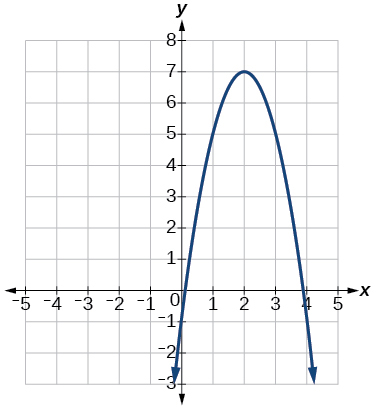$$f(x)=−2x^2+8x−1$$

62)63)$$f(x)=\dfrac{1}{2}x^2−3x+\dfrac{7}{2}$$

64)#### Numeric

For the exercises 65-69, use the table of values that represent points on the graph of a quadratic function. By determining the vertex and axis of symmetry, find the general form of the equation of the quadratic function.

65)

 $$x$$ $$y$$ –2 –1 0 1 2 5 2 1 2 5

$$f(x)=x^2+1$$

66)

 $$x$$ $$y$$ –2 –1 0 1 2 1 0 1 4 9

67)

 $$x$$ $$y$$ –2 –1 0 1 2 –2 1 2 1 –2

$$f(x)=2−x^2$$

68)

 $$x$$ $$y$$ –2 –1 0 1 2 –8 –3 0 1 0

69)

 $$x$$ $$y$$ –2 –1 0 1 2 8 2 0 2 8

$$f(x)=2x^2$$

#### Technology

For the exercises 70-74, use a calculator to find the answer.

70) Graph on the same set of axes the functions $$f(x)=x^2,f(x)=2x^2,$$ and $$f(x)=\dfrac{1}{3}x^2$$. What appears to be the effect of changing the coefficient?

71) Graph on the same set of axes $$f(x)=x^2,f(x)=x^2+2$$ and $$f(x)=x^2,f(x)=x^2+5$$ and $$f(x)=x^2−3$$. What appears to be the effect of adding a constant?

The graph is shifted up or down (a vertical shift).

72) Graph on the same set of axes $$f(x)=x^2,f(x)=(x−2)^2,f(x−3)2,$$ and $$f(x)=(x+4)^2$$. What appears to be the effect of adding or subtracting those numbers?

73) The path of an object projected at a $$45$$ degree angle with initial velocity of $$80$$ feet per second is given by the function $$h(x)=−\dfrac{32}{(80)^2}x^2+x$$ where $$x$$ is the horizontal distance traveled and $$h(x)$$ is the height in feet. Use the TRACE feature of your calculator to determine the height of the object when it has traveled $$100$$ feet away horizontally.

$$50$$ feet

74) A suspension bridge can be modeled by the quadratic function $$h(x)=.0001x^2$$ with $$−2000≤x≤2000$$ where $$|x|$$ is the number of feet from the center and $$h(x)$$ is height in feet. Use the TRACE feature of your calculator to estimate how far from the center does the bridge have a height of $$100$$ feet.

#### Extensions

For the exercises 75-78, use the vertex of the graph of the quadratic function and the direction the graph opens to find the domain and range of the function.

75) Vertex $$(1,−2)$$, opens up.

Domain is $$(−∞,∞)$$. Range is $$[−2,∞)$$.

76) Vertex $$(−1,2)$$ opens down.

77) Vertex $$(−5,11)$$, opens down.

Domain is $$(−∞,∞)$$ Range is $$(−∞,11]$$.

78) Vertex $$(−100,100)$$, opens up.

For the exercises 79-84, write the equation of the quadratic function that contains the given point and has the same shape as the given function.

79) Contains $$(1,1)$$ and has shape of $$f(x)=2x^2$$. Vertex is on the $$y$$-axis.

$$f(x)=2x^2−1$$

80) Contains $$(−1,4)$$ and has the shape of $$f(x)=2x^2$$. Vertex is on the $$y$$-axis.

81) Contains $$(2,3)$$ and has the shape of $$f(x)=3x^2$$. Vertex is on the $$y$$-axis.

$$f(x)=3x^2−9$$

82) Contains $$(1,−3)$$ and has the shape of $$f(x)=−x^2$$. Vertex is on the $$y$$-axis.

83) Contains $$(4,3)$$ and has the shape of $$f(x)=5x^2$$. Vertex is on the $$y$$-axis.

$$f(x)=5x^2−77$$

84) Contains $$(1,−6)$$ has the shape of $$f(x)=3x^2$$. Vertex has $$x$$-coordinate of $$-1$$.

#### Real-World Applications

85) Find the dimensions of the rectangular corral producing the greatest enclosed area given $$200$$ feet of fencing.

$$50$$ feet by $$50$$ feet. Maximize $$f(x)=−x^2+100x$$.

86) Find the dimensions of the rectangular corral split into $$2$$ pens of the same size producing the greatest possible enclosed area given $$300$$ feet of fencing.

87) Find the dimensions of the rectangular corral producing the greatest enclosed area split into $$3$$ pens of the same size given $$500$$ feet of fencing.

$$125$$ feet by $$62.5$$ feet. Maximize $$f(x)=−2x^2+250x$$.

88) Among all of the pairs of numbers whose sum is $$6$$, find the pair with the largest product. What is the product?

89) Among all of the pairs of numbers whose difference is $$12$$, find the pair with the smallest product. What is the product?

$$6$$ and $$-$$6; product is $$-36$$; maximize $$f(x)=x^2+12x$$.

90) Suppose that the price per unit in dollars of a cell phone production is modeled by $$p=45−0.0125x$$, where $$x$$ is in thousands of phones produced, and the revenue represented by thousands of dollars is $$R=x⋅p$$. Find the production level that will maximize revenue.

91) A rocket is launched in the air. Its height, in meters above sea level, as a function of time, in seconds, is given by $$h(t)=−4.9t^2+229t+234$$. Find the maximum height the rocket attains.

$$2909.56$$ meters

92) A ball is thrown in the air from the top of a building. Its height, in meters above ground, as a function of time, in seconds, is given by $$h(t)=−4.9t^2+24t+8$$. How long does it take to reach maximum height?

93) A soccer stadium holds $$62,000$$ spectators. With a ticket price of $$\11$$, the average attendance has been $$26,000$$. When the price dropped to $$\9$$, the average attendance rose to $$31,000$$. Assuming that attendance is linearly related to ticket price, what ticket price would maximize revenue?

$$\10.70$$

94) A farmer finds that if she plants $$75$$ trees per acre, each tree will yield $$20$$ bushels of fruit. She estimates that for each additional tree planted per acre, the yield of each tree will decrease by $$3$$ bushels. How many trees should she plant per acre to maximize her harvest?

## 3.3 Power Functions and Polynomial Functions

#### Verbal

1) Explain the difference between the coefficient of a power function and its degree.

The coefficient of the power function is the real number that is multiplied by the variable raised to a power. The degree is the highest power appearing in the function.

2) If a polynomial function is in factored form, what would be a good first step in order to determine the degree of the function?

3) In general, explain the end behavior of a power function with odd degree if the leading coefficient is positive.

As $$x$$ decreases without bound, so does $$f(x)$$. As $$x$$ increases without bound, so does $$f(x)$$.

4) What is the relationship between the degree of a polynomial function and the maximum number of turning points in its graph?

5) What can we conclude if, in general, the graph of a polynomial function exhibits the following end behavior? As $$x \rightarrow-\infty, f(x) \rightarrow-\infty$$ and as $$x \rightarrow \infty, f(x) \rightarrow-\infty$$.

The polynomial function is of even degree and leading coefficient is negative.

#### Algebraic

For the exercises 6-11, identify the function as a power function, a polynomial function, or neither.

6) $$f(x)=x^5$$

7) $$f(x)=(x^2)^3$$

Power function

8) $$f(x)=x−x^4$$

9) $$f(x)=\dfrac{x^2}{x^2−1}$$

Neither

10) $$f(x)=2x(x+2)(x−1)^2$$

11) $$f(x)=3^{x+1}$$

Neither

For the exercises 12-16, find the degree and leading coefficient for the given polynomial.

12) $$−3x^4$$

13) $$7−2x^2$$

Degree = $$2$$, Coefficient = $$-2$$

14) $$−2x^2− 3x^5+ x−6$$

15) $$x(4−x^2)(2x+1)$$

Degree =$$4$$, Coefficient = $$-2$$

16) $$x^2(2x−3)^2$$

For the exercises 17-24, determine the end behavior of the functions.

17) $$f(x)=x^4$$

As $$x→∞$$, $$f(x)→∞$$, as $$x→−∞$$, $$f(x)→∞$$

18) $$f(x)=x^3$$

19) $$f(x)=−x^4$$

As $$x→−∞$$, $$f(x)→−∞$$, as $$x→∞$$, $$f(x)→−∞$$

20) $$f(x)=−x^9$$

21) $$f(x)=−2x^4− 3x^2+ x−1$$

As $$x→−∞$$, $$f(x)→−∞$$, as $$x→∞$$, $$f(x)→−∞$$

22) $$f(x)=3x^2+ x−2$$

23) $$f(x)=x^2(2x^3−x+1)$$

As $$x→∞$$, $$f(x)→∞$$, as $$x→−∞$$, $$f(x)→−∞$$

24) $$f(x)=(2−x)^7$$

For the exercises 25-30, find the intercepts of the functions.

25) $$f(t)=2(t−1)(t+2)(t−3)$$

$$y$$-intercept is $$(0,12)$$, $$t$$-intercepts are $$(1,0);(–2,0);$$ and $$(3,0)$$.

26) $$g(n)=−2(3n−1)(2n+1)$$

27) $$f(x)=x^4−16$$

$$y$$-intercept is $$(0,−16)$$, $$x$$-intercepts are $$(2,0)$$ and $$(−2,0)$$.

28) $$f(x)=x^3+27$$

29) $$f(x)=x(x^2−2x−8)$$

$$y$$-intercept is $$(0,0)$$, $$x$$-intercepts are $$(0,0),(4,0),$$ and $$(−2, 0)$$.

30) $$f(x)=(x+3)(4x^2−1)$$

#### Graphical

For the exercises 31-38, determine the least possible degree of the polynomial function shown.

31)$$3$$

32)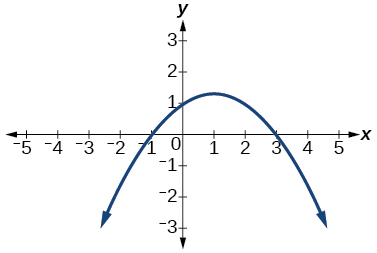33)$$5$$

34)35)$$3$$

36)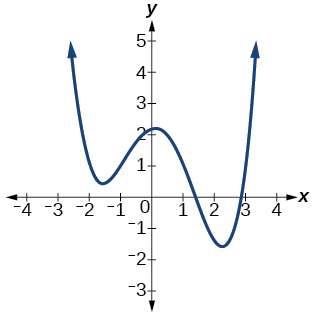37)$$5$$

38)For the exercises 39-45, determine whether the graph of the function provided is a graph of a polynomial function. If so, determine the number of turning points and the least possible degree for the function.

39)Yes. Number of turning points is $$2$$. Least possible degree is $$3$$.

40)41)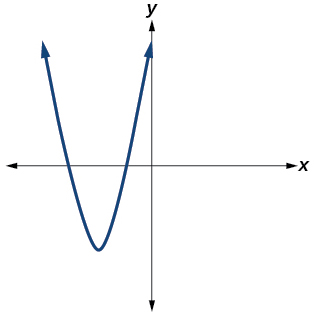Yes. Number of turning points is $$1$$. Least possible degree is $$2$$.

42)43)Yes. Number of turning points is $$0$$. Least possible degree is $$1$$.

44)No.

45)Yes. Number of turning points is $$0$$. Least possible degree is $$1$$.

#### Numeric

For the exercises 46-50, make a table to confirm the end behavior of the function.

46) $$f(x)=−x^3$$

47) $$f(x)=x^4−5x^2$$

$$x$$ $$f(x)$$
10 9,500
100 99,950,000
–10 9,500
–100 99,950,000

as $$x→−∞,$$ $$f(x)→∞$$, as $$x→∞,$$ $$f(x)→∞$$

48) $$f(x)=x^2(1−x)^2$$

49) $$f(x)=(x−1)(x−2)(3−x)$$

$$x$$ $$f(x)$$
10 –504
100 –941,094
–10 1,716
–100 1,061,106

as $$x→−∞,$$ $$f(x)→∞$$, as $$x→∞,$$ $$f(x)→−∞$$

50) $$f(x)=\dfrac{x^5}{10}−x^4$$

#### Technology

For the exercises 51-60, graph the polynomial functions using a calculator. Based on the graph, determine the intercepts and the end behavior.

51) $$f(x)=x^3(x−2)$$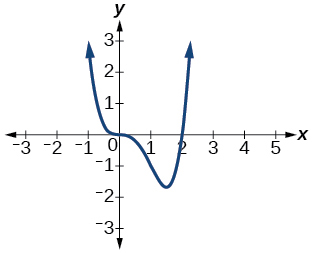The $$y$$-intercept is $$(0, 0)$$. The x-intercepts are $$(0, 0), (2, 0).$$ As $$x→−∞,$$ $$f(x)→∞$$, as $$x→∞,$$ $$f(x)→∞$$

52) $$f(x)=x(x−3)(x+3)$$

53) $$f(x)=x(14−2x)(10−2x)$$The $$y$$-intercept is $$(0,0)$$. The x-intercepts are $$(0, 0), (5, 0), (7, 0)$$. As $$x→−∞$$, $$f(x)→−∞$$, as $$x→∞,$$ $$f(x)→∞$$

54) $$f(x)=x(14−2x)(10−2x)^2$$

55) $$f(x)=x^3−16x$$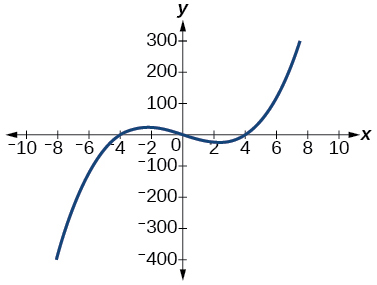The $$y$$-intercept is $$(0, 0)$$. The x-intercept is $$(−4, 0), (0, 0), (4, 0)$$. As $$x→−∞$$, $$f(x)→−∞$$, as $$x→∞,$$ $$f(x)→∞$$

56) $$f(x)=x^3−27$$

57) $$f(x)=x^4−81$$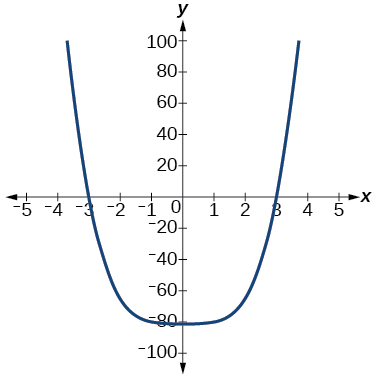The $$y$$-intercept is $$(0, -81)$$. The $$x$$-intercept are $$(3, 0), (−3, 0)$$. As $$x→−∞,$$ $$f(x)→∞$$, as $$x→∞,$$ $$f(x)→∞$$

58) $$f(x)=−x^3+x^2+2x$$

59) $$f(x)=x^3−2x^2−15x$$The $$y$$-intercept is $$(0, 0)$$. The x-intercepts are $$(−3, 0), (0, 0), (5, 0).$$ As $$x→−∞$$, $$f(x)→−∞$$, as $$x→∞,$$ $$f(x)→∞$$

60) $$f(x)=x^3−0.01x$$

#### Extensions

For the exercises 61-65, use the information about the graph of a polynomial function to determine the function. Assume the leading coefficient is $$1$$ or $$-1$$. There may be more than one correct answer.

61) The $$y$$-intercept is $$(0,−4)$$. The $$x$$-intercepts are $$(−2,0), (2,0)$$. Degree is $$2$$.

End behavior: as $$x→−∞,$$ $$f(x)→∞$$, as $$x→∞,$$ $$f(x)→∞$$.

$$f(x)=x^2−4$$

62) The $$y$$-intercept is $$(0,9)$$. The $$x$$-intercepts are $$(−3,0), (3,0)$$. Degree is $$2$$.

End behavior: as $$x→−∞,$$ $$f(x)→−∞$$, as $$x→∞,$$ $$f(x)→−∞$$.

63) The $$y$$-intercept is $$(0,0)$$. The $$x$$-intercepts are $$(0,0), (2,0)$$. Degree is $$3$$.

End behavior: as $$x→−∞,$$ $$f(x)→−∞$$, as $$x→∞,$$ $$f(x)→∞$$.

$$f(x)=x^3−4x^2+4x$$

64) The $$y$$-intercept is $$(0,1)$$. The x-intercept is $$(1,0)$$. Degree is $$3$$.

End behavior: as $$x→−∞$$, $$f(x)→∞$$, as $$x→∞$$, $$f(x)→−∞$$.

65) The $$y$$-intercept is $$(0,1)$$. There is no $$x$$-intercept. Degree is $$4$$.

End behavior: as $$x→−∞,$$ $$f(x)→∞$$, as $$x→∞,$$ $$f(x)→∞$$.

$$f(x)=x^4+1$$

#### Real-World Applications

For the exercises 66-70, use the written statements to construct a polynomial function that represents the required information.

66) An oil slick is expanding as a circle. The radius of the circle is increasing at the rate of $$20$$ meters per day. Express the area of the circle as a function of $$d$$, the number of days elapsed.

67) A cube has an edge of $$3$$ feet. The edge is increasing at the rate of $$2$$ feet per minute. Express the volume of the cube as a function of $$m$$, the number of minutes elapsed.

$$V(m)=8m^3+36m^2+54m+27$$

68) A rectangle has a length of $$10$$ inches and a width of $$6$$ inches. If the length is increased by $$x$$ inches and the width increased by twice that amount, express the area of the rectangle as a function of $$x$$.

69) An open box is to be constructed by cutting out square corners of $$x$$-inch sides from a piece of cardboard $$8$$ inches by $$8$$ inches and then folding up the sides. Express the volume of the box as a function of $$x$$.

$$V(x)=4x^3−32x^2+64x$$

70) A rectangle is twice as long as it is wide. Squares of side $$2$$ feet are cut out from each corner. Then the sides are folded up to make an open box. Express the volume of the box as a function of the width ($$x$$).

## 3.4 Graphs of Polynomial Functions

#### Verbal

1) What is the difference between an $$x$$-intercept and a zero of a polynomial function $$f$$?

The $$x$$-intercept is where the graph of the function crosses the $$x$$-axis, and the zero of the function is the input value for which $$f(x)=0$$.

2) If a polynomial function of degree $$n$$ has $$n$$ distinct zeros, what do you know about the graph of the function?

3) Explain how the Intermediate Value Theorem can assist us in finding a zero of a function.

If we evaluate the function at $$a$$ and at $$b$$ and the sign of the function value changes, then we know a zero exists between $$a$$ and $$b$$.

4) Explain how the factored form of the polynomial helps us in graphing it.

5) If the graph of a polynomial just touches the $$x$$-axis and then changes direction, what can we conclude about the factored form of the polynomial?

There will be a factor raised to an even power.

#### Algebraic

For the exercises 6-23, find the $$x$$-or $$t$$-intercepts of the polynomial functions.

6) $$C(t)=2(t−4)(t+1)(t−6)$$

7) $$C(t)=3(t+2)(t−3)(t+5)$$

$$(−2,0),(3,0),(−5,0)$$

8) $$C(t)=4t(t−2)^2(t+1)$$

9) $$C(t)=2t(t−3)(t+1)^2$$

$$(3,0),(−1,0),(0,0)$$

10) $$C(t)=2t^4−8t^3+6t^2$$

11) $$C(t)=4t^4+12t^3−40t^2$$

$$(0,0), (−5,0), (2,0)$$

12) $$f(x)=x^4−x^2$$

13) $$f(x)=x^3+x^2−20x$$

$$(0,0), (−5,0), (4,0)$$

14) $$f(x)=x^3+6x^2−7x$$

15) $$f(x)=x^3+x^2−4x−4$$

$$(2,0), (−2,0), (−1,0)$$

16) $$f(x)=x^3+2x^2−9x−18$$

17) $$f(x)=2x^3−x^2−8x+4$$

$$(−2,0),(2,0),\left(\dfrac{1}{2},0\right)$$

18) $$f(x)=x^6−7x^3−8$$

19) $$f(x)=2x^4+6x^2−8$$

$$(1,0), (−1,0)$$

20) $$f(x)=x^3−3x^2−x+3$$

21) $$f(x)=x^6−2x^4−3x^2$$

$$(0,0),(\sqrt{3},0),(−\sqrt{3},0)$$

22) $$f(x)=x^6−3x^4−4x^2$$

23) $$f(x)=x^5−5x^3+4x$$

$$(0,0), (1,0), (−1,0), (2,0), (−2,0)$$

For the exercises 24-29, use the Intermediate Value Theorem to confirm that the given polynomial has at least one zero within the given interval.

24) $$f(x)=x^3−9x$$, between $$x=−4$$ and $$x=−2$$.

25) $$f(x)=x^3−9x$$, between $$x=2$$ and $$x=4$$.

$$f(2)=–10$$ and $$f(4)=28$$. Sign change confirms.

26) $$f(x)=x^5−2x$$, between $$x=1$$ and $$x=2$$.

27) $$f(x)=−x^4+4$$, between $$x=1$$ and $$x=3$$.

$$f(1)=3$$ and $$f(3)=–77.$$ Sign change confirms.

28) $$f(x)=−2x^3−x$$, between $$x=–1$$ and $$x=1$$.

29) $$f(x)=x^3−100x+2$$, between $$x=0.01$$ and $$x=0.1$$

$$f(0.01)=1.000001$$ and $$f(0.1)=–7.999$$. Sign change confirms.

For the exercises 30-41, find the zeros and give the multiplicity of each.

30) $$f(x)=(x+2)^3(x−3)^2$$

31) $$f(x)=x^2(2x+3)^5(x−4)^2$$

$$0$$ with multiplicity $$2$$, $$−\dfrac{3}{2}$$ with multiplicity $$5$$, $$4$$ with multiplicity $$2$$

32) $$f(x)=x^3(x−1)^3(x+2)$$

33) $$f(x)=x^2(x^2+4x+4)$$

$$0$$ with multiplicity $$2$$, $$-2$$ with multiplicity $$2$$

34) $$f(x)=(2x+1)^3(9x^2−6x+1)$$

35) $$f(x)=(3x+2)^5(x^2−10x+25)$$

$$−\dfrac{2}{3}$$ with multiplicity $$5$$, $$5$$ with multiplicity $$2$$

36) $$f(x)=x(4x^2−12x+9)(x^2+8x+16)$$

37) $$f(x)=x^6−x^5−2x^4$$

$$0$$ with multiplicity $$4$$, $$2$$ with multiplicity $$1$$, $$-1$$ with multiplicity $$1$$

38) $$f(x)=3x^4+6x^3+3x^2$$

39) $$f(x)=4x^5−12x^4+9x^3$$

$$\dfrac{3}{2}$$ with multiplicity $$2$$, $$0$$ with multiplicity $$3$$

40) $$f(x)=2x^4(x^3−4x^2+4x)$$

41) $$f(x)=4x^4(9x^4−12x^3+4x^2)$$

$$0$$ with multiplicity $$6$$, $$\dfrac{2}{3}$$ with multiplicity $$2$$

#### Graphical

For the exercises 42-47, graph the polynomial functions. Note $$x$$- and $$y$$- intercepts, multiplicity, and end behavior.

42) $$f(x)=(x+3)^2(x−2)$$

43) $$g(x)=(x+4)(x−1)^2$$

$$x$$-intercepts, $$(1, 0)$$ with multiplicity $$2$$, $$(–4, 0)$$ with multiplicity $$1$$, $$y$$- intercept $$(0, 4)$$. As $$x→−∞$$, $$f(x)→−∞$$, as $$x→∞,$$ $$f(x)→∞$$.44) $$h(x)=(x−1)^3(x+3)^2$$

45) $$k(x)=(x−3)^3(x−2)^2$$

$$x$$-intercepts $$(3,0)$$ with multiplicity $$3$$, $$(2,0)$$ with multiplicity $$2$$, $$y$$- intercept $$(0,–108).$$ As $$x→−∞,$$ $$f(x)→−∞$$, as $$x→∞,$$ $$f(x)→∞$$.46) $$m(x)=−2x(x−1)(x+3)$$

47) $$n(x)=−3x(x+2)(x−4)$$

$$x$$-intercepts $$(0, 0),(–2, 0),(4, 0)$$ with multiplicity $$1$$, $$y$$-intercept (0, 0). As $$x→−∞,$$ $$f(x)→∞$$, as $$x→∞,$$ $$f(x)→−∞$$.For the exercises 48-52, use the graphs to write the formula for a polynomial function of least degree.

48)49)$$f(x)=−\dfrac{2}{9}(x−3)(x+1)(x+3)$$

50)51)$$f(x)=\dfrac{1}{4}(x+2)^2(x−3)$$

52)For the exercises 53-56, use the graph to identify zeros and multiplicity.

53)$$–4, –2, 1, 3$$ with multiplicity $$1$$

54)55)$$–2, 3$$ each with multiplicity $$2$$

56)For the exercises 57-66, use the given information about the polynomial graph to write the equation.

57) Degree $$3$$. Zeros at $$x=–2$$,$$x=1$$, and $$x=3$$. $$y$$-intercept at $$(0,–4)$$

$$f(x)=−\dfrac{2}{3}(x+2)(x−1)(x−3)$$

58) Degree $$3$$. Zeros at $$x=–5$$, $$x=–2$$, and $$x=1$$. $$y$$-intercept at $$(0,6)$$

59) Degree $$5$$. Roots of multiplicity $$2$$ at $$x=3$$ and $$x=1$$, and a root of multiplicity $$1$$ at $$x=–3$$. $$y$$-intercept at $$(0,9)$$

$$f(x)=\dfrac{1}{3}(x−3)^2(x−1)^2(x+3)$$

60) Degree $$4$$. Root of multiplicity $$2$$ at $$x=4$$, and a roots of multiplicity $$1$$ at $$x=1$$ and $$x=–2$$. $$y$$-intercept at $$(0,–3)$$

61) Degree $$5$$. Double zero at $$x=1$$, and triple zero at $$x=3$$. Passes through the point $$(2,15)$$

$$f(x)=−15(x−1)^2(x−3)^3$$

62) Degree $$3$$. Zeros at $$x=4$$, $$x=3$$, and $$x=2$$. $$y$$-intercept at $$(0,−24)$$

63) Degree $$3$$. Zeros at $$x=−3$$, $$x=−2$$ and $$x=1$$. $$y$$-intercept at $$(0,12)$$

$$f(x)=−2(x+3)(x+2)(x−1)$$

64) Degree $$5$$. Roots of multiplicity $$2$$ at $$x=−3$$ and $$x=2$$ and a root of multiplicity $$1$$ at $$x=−2$$. $$y$$-intercept at $$(0, 4)$$.

65) Degree $$4$$. Roots of multiplicity $$2$$ at $$x=\dfrac{1}{2}$$ and roots of multiplicity $$1$$ at $$x=6$$ and $$x=−2$$. $$y$$-intercept at $$(0,18)$$

$$f(x)=−\dfrac{3}{2}(2x−1)^2(x−6)(x+2)$$

66) Double zero at $$x=−3$$ and triple zero at $$x=0$$. Passes through the point $$(1,32)$$.

#### Technology

For the exercises 67-71, use a calculator to approximate local minima and maxima or the global minimum and maximum.

67) $$f(x)=x^3−x−1$$

local max $$(–.58, –.62)$$, local min $$(.58, –1.38)$$

68) $$f(x)=2x^3−3x−1$$

69) $$f(x)=x^4+x$$

global min $$(–.63, –.47)$$

70) $$f(x)=−x^4+3x−2$$

71) $$f(x)=x^4−x^3+1$$

global min $$(.75, .89)$$

#### Extensions

For the exercises 72-74, use the graphs to write a polynomial function of least degree.

72)73)$$f(x)=(x−500)^2(x+200)$$

74)#### Real-World Applications

For the exercises 75-, write the polynomial function that models the given situation.

75) A rectangle has a length of $$10$$ units and a width of 8 units. Squares of $$x$$ by $$x$$ units are cut out of each corner, and then the sides are folded up to create an open box. Express the volume of the box as a polynomial function in terms of $$x$$.

$$f(x)=4x^3−36x^2+80x$$

76) Consider the same rectangle of the preceding problem. Squares of $$2x$$ by $$2x$$ units are cut out of each corner. Express the volume of the box as a polynomial in terms of $$x$$.

77) A square has sides of $$12$$ units. Squares $$x +1$$ by $$x +1$$ units are cut out of each corner, and then the sides are folded up to create an open box. Express the volume of the box as a function in terms of $$x$$.

$$f(x)=4x^3−36x^2+60x+100$$

78) A cylinder has a radius of $$x+2$$ units and a height of $$3$$ units greater. Express the volume of the cylinder as a polynomial function.

79) A right circular cone has a radius of $$3x+6$$ and a height $$3$$ units less. Express the volume of the cone as a polynomial function. The volume of a cone is $$V=\dfrac{1}{3}πr^2h$$ for radius $$r$$ and height $$h$$.

$$f(x)=π(9x^3+45x^2+72x+36)$$

## 3.5 Dividing Polynomials

#### Verbal

1) If division of a polynomial by a binomial results in a remainder of zero, what can be conclude?

The binomial is a factor of the polynomial.

2) If a polynomial of degree $$n$$ is divided by a binomial of degree $$1$$, what is the degree of the quotient?

#### Algebraic

For the exercises 3-13, use long division to divide. Specify the quotient and the remainder.

3) $$(x^2+5x−1)÷(x−1)$$

$$\mathrm{x+6+\dfrac{5}{x−1}, quotient: x+6, remainder: 5}$$

4) $$(2x^2−9x−5)÷(x−5)$$

5) $$(3x^2+23x+14)÷(x+7)$$

$$\mathrm{3x+2, quotient: 3x+2, remainder: 0}$$

6) $$(4x^2−10x+6)÷(4x+2)$$

7) $$(6x^2−25x−25)÷(6x+5)$$

$$\mathrm{x−5, quotient: x−5, remainder: 0}$$

8) $$(−x^2−1)÷(x+1)$$

9) $$(2x^2−3x+2)÷(x+2)$$

$$\mathrm{2x−7+\dfrac{16}{x+2}, quotient: 2x−7, remainder: 16}$$

10) $$(x^3−126)÷(x−5)$$

11) $$(3x^2−5x+4)÷(3x+1)$$

$$\mathrm{x−2+\dfrac{6}{3x+1}, quotient: x−2, remainder: 6}$$

12) $$(x^3−3x^2+5x−6)÷(x−2)$$

13) $$(2x^3+3x^2−4x+15)÷(x+3)$$

$$\mathrm{2x^2−3x+5, quotient: 2x^2−3x+5, remainder: 0}$$

For the exercises 14-37, use synthetic division to find the quotient.

14) $$(3x^3−2x^2+x−4)÷(x+3)$$

15) $$(2x^3−6x^2−7x+6)÷(x−4)$$

$$2x^2+2x+1+\dfrac{10}{x−4}$$

16) $$(6x^3−10x^2−7x−15)÷(x+1)$$

17) $$(4x^3−12x^2−5x−1)÷(2x+1)$$

$$2x^2−7x+1−\dfrac{2}{2x+1}$$

18) $$(9x^3−9x^2+18x+5)÷(3x−1)$$

19) $$(3x^3−2x^2+x−4)÷(x+3)$$

$$3x^2−11x+34−\dfrac{106}{x+3}$$

20) $$(−6x^3+x^2−4)÷(2x−3)$$

21) $$(2x^3+7x^2−13x−3)÷(2x−3)$$

$$x^2+5x+1$$

22) $$(3x^3−5x^2+2x+3)÷(x+2)$$

23) $$(4x^3−5x^2+13)÷(x+4)$$

$$4x^2−21x+84−\dfrac{323}{x+4}$$

24) $$(x^3−3x+2)÷(x+2)$$

25) $$(x^3−21x^2+147x−343)÷(x−7)$$

$$x^2−14x+49$$

26) $$(x^3−15x^2+75x−125)÷(x−5)$$

27) $$(9x^3−x+2)÷(3x−1)$$

$$3x^2+x+\dfrac{2}{3x−1}$$

28) $$(6x^3−x^2+5x+2)÷(3x+1)$$

29) $$(x^4+x^3−3x^2−2x+1)÷(x+1)$$

$$x^3−3x+1$$

30) $$(x^4−3x^2+1)÷(x−1)$$

31) $$(x^4+2x^3−3x^2+2x+6)÷(x+3)$$

$$x^3−x^2+2$$

32) $$(x^4−10x^3+37x^2−60x+36)÷(x−2)$$

33) $$(x^4−8x^3+24x^2−32x+16)÷(x−2)$$

$$x^3−6x^2+12x−8$$

34) $$(x^4+5x^3−3x^2−13x+10)÷(x+5)$$

35) $$(x^4−12x^3+54x^2−108x+81)÷(x−3)$$

$$x^3−9x^2+27x−27$$

36) $$(4x^4−2x^3−4x+2)÷(2x−1)$$

37) $$(4x^4+2x^3−4x^2+2x+2)÷(2x+1)$$

$$2x^3−2x+2$$

For the exercises 38-43, use synthetic division to determine whether the first expression is a factor of the second. If it is, indicate the factorization.

38) $$x−2, 4x^3−3x^2−8x+4$$

39) $$x−2, 3x^4−6x^3−5x+10$$

Yes $$(x−2)(3x^3−5)$$

40) $$x+3, −4x^3+5x^2+8$$

41) $$x−2, 4x^4−15x^2−4$$

Yes $$(x−2)(4x^3+8x^2+x+2)$$

42) $$x−\dfrac{1}{2}, 2x^4−x^3+2x−1$$

43) $$x+\dfrac{1}{3}, 3x^4+x^3−3x+1$$

No

#### Graphical

For the exercises 44-48, use the graph of the third-degree polynomial and one factor to write the factored form of the polynomial suggested by the graph. The leading coefficient is one.

44) Factor is $$x^2−x+3$$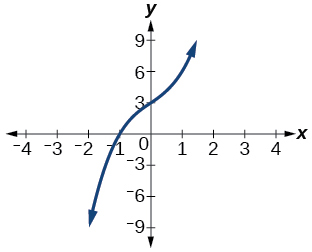45) Factor is $$(x^2+2x+4)$$$$(x−1)(x^2+2x+4)$$

46) Factor is $$x^2+2x+5$$47) Factor is $$x^2+x+1$$$$(x−5)(x^2+x+1)$$

48) Factor is $$x^2+2x+2$$For the exercises 49-53, use synthetic division to find the quotient and remainder.

49) $$\dfrac{4x^3−33}{x−2}$$

$$\mathrm{Quotient: 4x^2+8x+16, remainder: −1}$$

50) $$\dfrac{2x^3+25}{x+3}$$

51) $$\dfrac{3x^3+2x−5}{x−1}$$

$$\mathrm{Quotient: 3x^2+3x+5, remainder: 0}$$

52) $$\dfrac{−4x^3−x^2−12}{x+4}$$

53) $$\dfrac{x^4−22}{x+2}$$

$$\mathrm{Quotient: x^3−2x^2+4x−8, remainder: −6}$$

#### Technology

For the exercises 54-58, use a calculator with CAS to answer the questions.

54) Consider $$\dfrac{x^k−1}{x−1}$$ with $$k=1, 2, 3$$. What do you expect the result to be if $$k=4$$?

55) Consider $$\dfrac{x^k+1}{x+1}$$ for $$k=1, 3, 5$$. What do you expect the result to be if $$k=7$$?

$$x^6−x^5+x^4−x^3+x^2−x+1$$

56) Consider $$\dfrac{x^4−k^4}{x−k}$$ for $$k=1, 2, 3$$. What do you expect the result to be if $$k=4$$?

57) Consider $$\dfrac{x^k}{x+1}$$ with $$k=1, 2, 3$$. What do you expect the result to be if $$k=4$$?

$$x^3−x^2+x−1+\dfrac{1}{x+1}$$

58) Consider $$\dfrac{x^k}{x−1}$$ with $$k=1, 2, 3$$. What do you expect the result to be if $$k=4$$?

#### Extensions

For the exercises 59-63, use synthetic division to determine the quotient involving a complex number.

59) $$\dfrac{x+1}{x−i}$$

$$1+\dfrac{1+i}{x−i}$$

60) $$\dfrac{x^2+1}{x−i}$$

61) $$\dfrac{x+1}{x+i}$$

$$1+\dfrac{1−i}{x+i}$$

62) $$\dfrac{x^2+1}{x+i}$$

63) $$\dfrac{x^3+1}{x−i}$$

$$x^2−ix−1+\dfrac{1−i}{x−i}$$

#### Real-World Applications

For the exercises 64-66, use the given length and area of a rectangle to express the width algebraically.

64) Length is $$x+5$$, area is $$2x^2+9x−5$$.

65) Length is $$2x + 5$$, area is $$4x^3+10x^2+6x+15$$.

$$2x^2+3$$

66) Length is $$3x–4$$, area is $$6x^4−8x^3+9x^2−9x−4$$.

For the exercises 67-70, use the given volume of a box and its length and width to express the height of the box algebraically.

67) Volume is $$12x^3+20x^2−21x−36$$, length is $$2x+3$$, width is $$3x−4$$.

$$2x+3$$

68) Volume is $$18x^3−21x^2−40x+48$$, length is $$3x–4$$, width is $$3x–4$$.

69) Volume is $$10x^3+27x^2+2x−24$$, length is $$5x–4$$, width is $$2x+3$$.

$$x+2$$

70) Volume is $$10x^3+30x^2−8x−24$$, length is $$2$$, width is $$x+3$$.

For the exercises 71-73, use the given volume and radius of a cylinder to express the height of the cylinder algebraically.

71) Volume is $$π(25x^3−65x^2−29x−3)$$, radius is $$5x+1$$.

$$x−3$$

72) Volume is $$π(4x^3+12x^2−15x−50)$$, radius is $$2x+5$$.

73) Volume is $$π(3x^4+24x^3+46x^2−16x−32)$$, radius is $$x+4$$.

$$3x^2−2$$

## 3.6 Zeros of Polynomial Functions

#### Verbal

1) Describe a use for the Remainder Theorem.

The theorem can be used to evaluate a polynomial.

2) Explain why the Rational Zero Theorem does not guarantee finding zeros of a polynomial function.

3) What is the difference between rational and real zeros?

Rational zeros can be expressed as fractions whereas real zeros include irrational numbers.

4) If Descartes’ Rule of Signs reveals a no change of signs or one sign of changes, what specific conclusion can be drawn?

5) If synthetic division reveals a zero, why should we try that value again as a possible solution?

Polynomial functions can have repeated zeros, so the fact that number is a zero doesn’t preclude it being a zero again.

#### Algebraic

For the exercises 6-13, use the Remainder Theorem to find the remainder.

6) $$(x^4−9x^2+14)÷(x−2)$$

7) $$(3x^3−2x^2+x−4)÷(x+3)$$

$$−106$$

8) $$(x^4+5x^3−4x−17)÷(x+1)$$

9) $$(−3x^2+6x+24)÷(x−4)$$

$$0$$

10) $$(5x^5−4x^4+3x^3−2x^2+x−1)÷(x+6)$$

11) $$(x^4−1)÷(x−4)$$

$$255$$

12) $$(3x^3+4x^2−8x+2)÷(x−3)$$

13) $$(4x^3+5x^2−2x+7)÷(x+2)$$

$$−1$$

For the exercises 14-21, use the Factor Theorem to find all real zeros for the given polynomial function and one factor.

14) $$f(x)=2x^3−9x^2+13x−6; x−1$$

15) $$f(x)=2x^3+x^2−5x+2; x+2$$

$$−2, 1, \dfrac{1}{2}$$

16) $$f(x)=3x^3+x^2−20x+12; x+3$$

17) $$f(x)=2x^3+3x^2+x+6;x+2$$

$$−2$$

18) $$f(x)=−5x^3+16x^2−9;x−3$$

19) $$x^3+3x^2+4x+12;x+3$$

$$−3$$

20) $$4x^3−7x+3;x−1$$

21) $$2x^3+5x^2−12x−30,2x+5$$

$$−\dfrac{5}{2}, \sqrt{6}, −\sqrt{6}$$

For the exercises 22-39, use the Rational Zero Theorem to find all real zeros.

22) $$x^3−3x^2−10x+24=0$$

23) $$2x^3+7x^2−10x−24=0$$

$$2, −4, −\dfrac{3}{2}$$

24) $$x^3+2x^2−9x−18=0$$

25) $$x^3+5x^2−16x−80=0$$

$$4, −4, −5$$

26) $$x^3−3x^2−25x+75=0$$

27) $$2x^3−3x^2−32x−15=0$$

$$5, −3, −\dfrac{1}{2}$$

28) $$2x^3+x^2−7x−6=0$$

29) $$2x^3−3x^2−x+1=0$$

$$\dfrac{1}{2}, \dfrac{1+\sqrt{5}}{2}, \dfrac{1−\sqrt{5}}{2}$$

30) $$3x^3−x^2−11x−6=0$$

31) $$2x^3−5x^2+9x−9=0$$

$$\dfrac{3}{2}$$

32) $$2x^3−3x^2+4x+3=0$$

33) $$x^4−2x^3−7x^2+8x+12=0$$

$$2, 3, −1, −2$$

34) $$x^4+2x^3−9x^2−2x+8=0$$

35) $$4x^4+4x^3−25x^2−x+6=0$$

$$\dfrac{1}{2}, −\dfrac{1}{2}, 2, −3$$

36) $$2x^4−3x^3−15x^2+32x−12=0$$

37) $$x^4+2x^3−4x^2−10x−5=0$$

$$−1, −1, \sqrt{5}, −\sqrt{5}$$

38) $$4x^3−3x+1=0$$

39) $$8x^4+26x^3+39x^2+26x+6$$

$$−\dfrac{3}{4}, −\dfrac{1}{2}$$

For the exercises 40-45, find all complex solutions (real and non-real).

40) $$x^3+x^2+x+1=0$$

41) $$x^3−8x^2+25x−26=0$$

$$2, 3+2i, 3−2i$$

42) $$x^3+13x^2+57x+85=0$$

43) $$3x^3−4x^2+11x+10=0$$

$$−\dfrac{2}{3}, 1+2i, 1−2i$$

44) $$x^4+2x^3+22x^2+50x−75=0$$

45) $$2x^3−3x^2+32x+17=0$$

$$−\dfrac{1}{2}, 1+4i, 1−4i$$

#### Graphical

For the exercises 46-55, use Descartes’ Rule to determine the possible number of positive and negative solutions. Confirm with the given graph.

46) $$f(x)=x^3−1$$

47) $$f(x)=x^4−x^2−1$$

$$1$$ positive, $$1$$ negative48) $$f(x)=x^3−2x^2−5x+6$$

49) $$f(x)=x^3−2x^2+x−1$$

$$3$$ or $$1$$ positive, $$0$$ negative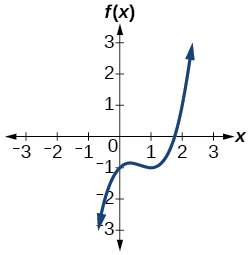50) $$f(x)=x^4+2x^3−12x^2+14x−5$$

51) $$f(x)=2x^3+37x^2+200x+300$$

$$0$$ positive, $$3$$ or $$1$$ negative52) $$f(x)=x^3−2x^2−16x+32$$

53) $$f(x)=2x^4−5x^3−5x^2+5x+3$$

$$2$$ or $$0$$ positive, $$2$$ or $$0$$ negative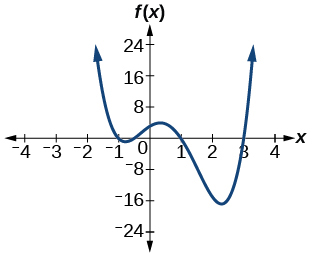54) $$f(x)=2x^4−5x^3−14x^2+20x+8$$

55) $$f(x)=10x^4−21x^2+11$$

$$2$$ or $$0$$ positive, $$2$$ or $$0$$ negative#### Numeric

For the exercises 56-60, list all possible rational zeros for the functions.

56) $$f(x)=x^4+3x^3−4x+4$$

57) $$f(x)=2x^3+3x^2−8x+5$$

$$±5, ±1, ± \dfrac{5}{2}$$

58) $$f(x)=3x^3+5x^2−5x+4$$

59) $$f(x)=6x^4−10x^2+13x+1$$

$$±1, ±\dfrac{1}{2}, ±\dfrac{1}{3}, ±\dfrac{1}{6}$$

60) $$f(x)=4x^5−10x^4+8x^3+x^2−8$$

#### Technology

For the exercises 61-65, use your calculator to graph the polynomial function. Based on the graph, find the rational zeros. All real solutions are rational.

61) $$f(x)=6x^3−7x^2+1$$

$$1, \dfrac{1}{2}, −\dfrac{1}{3}$$

62) $$f(x)=4x^3−4x^2−13x−5$$

63) $$f(x)=8x^3−6x^2−23x+6$$

$$2, \dfrac{1}{4}, −\dfrac{3}{2}$$

64) $$f(x)=12x^4+55x^3+12x^2−117x+54$$

65) $$f(x)=16x^4−24x^3+x^2−15x+25$$

$$\dfrac{5}{4}$$

#### Extensions

For the exercises 66-70, construct a polynomial function of least degree possible using the given information.

66) Real roots: $$–1, 1, 3$$ and $$(2,f(2))=(2,4)$$

67) Real roots: $$–1$$ (with multiplicity $$2$$ and $$1$$) and $$(2,f(2))=(2,4)$$

$$f(x)=\dfrac{4}{9}(x^3+x^2−x−1)$$

68) Real roots: $$–2, \dfrac{1}{2}$$ (with multiplicity $$2$$) and $$(−3,f(−3))=(−3,5)$$

69) Real roots:$$−\dfrac{1}{2}, 0,\dfrac{1}{2}$$ and $$(−2,f(−2))=(−2,6)$$

$$f(x)=−\dfrac{1}{5}(4x^3−x)$$

70) Real roots: $$–4, –1, 1, 4$$ and $$(−2,f(−2))=(−2,10)$$

#### Real-World Applications

For the exercises 71-75, find the dimensions of the box described.

71) The length is twice as long as the width. The height is $$2$$ inches greater than the width. The volume is $$192$$ cubic inches.

$$8$$ by $$4$$ by $$6$$ inches

72) The length, width, and height are consecutive whole numbers. The volume is $$120$$cubic inches.

73) The length is one inch more than the width, which is one inch more than the height. The volume is $$86.625$$ cubic inches.

$$5.5$$ by $$4.5$$ by $$3.5$$ inches

74) The length is three times the height and the height is one inch less than the width. The volume is $$108$$ cubic inches.

75) The length is $$3$$ inches more than the width. The width is $$2$$ inches more than the height. The volume is $$120$$ cubic inches.

$$8$$ by $$5$$ by $$3$$ inches

For the exercises 76-80, find the dimensions of the right circular cylinder described.

76) The radius is $$3$$ inches more than the height. The volume is $$16π$$ cubic meters.

77) The height is one less than one half the radius. The volume is $$72π$$ cubic meters.

Radius = $$6$$ meters, Height = $$2$$ meters

78) The radius and height differ by one meter. The radius is larger and the volume is $$48π$$ cubic meters.

79) The radius and height differ by two meters. The height is greater and the volume is $$28.125π$$ cubic meters.

Radius = $$2.5$$ meters, Height = $$4.5$$ meters

80) The radius is $$\dfrac{1}{3}$$ meter greater than the height. The volume is $$\dfrac{98}{9}π$$ cubic meters.

## 3.7 Rational Functions

#### Verbal

1) What is the fundamental difference in the algebraic representation of a polynomial function and a rational function?

The rational function will be represented by a quotient of polynomial functions.

2) What is the fundamental difference in the graphs of polynomial functions and rational functions?

3) If the graph of a rational function has a removable discontinuity, what must be true of the functional rule?

The numerator and denominator must have a common factor.

4) Can a graph of a rational function have no vertical asymptote? If so, how?

5) Can a graph of a rational function have no x-intercepts? If so, how?

Yes. The numerator of the formula of the functions would have only complex roots and/or factors common to both the numerator and denominator.

#### Algebraic

For the exercises 6-9, find the domain of the rational functions.

6) $$f(x)=\dfrac{x-1}{x+2}$$

7) $$f(x)=\dfrac{x+1}{x^{2}-1}$$

All reals $$x \neq -1,1$$

8) $$f(x)=\dfrac{x^{2}+4}{x^{2}-2 x-8}$$

9) $$f(x)=\dfrac{x^{2}+4 x-3}{x^{4}-5 x^{2}+4}$$

All reals $$x \neq-1,-2,1,2$$

For the exercises 10-19, find the domain, vertical asymptotes, and horizontal asymptotes of the functions.

10) $$f(x)=\dfrac{4}{x-1}$$

11) $$f(x)=\dfrac{2}{5 x+2}$$

V.A. at $$x=-\dfrac{2}{5}$$; H.A. at $$y=0$$; Domain is all reals $$x \neq-\dfrac{2}{5}$$

12) $$f(x)=\dfrac{x}{x^{2}-9}$$

13) $$f(x)=\dfrac{x}{x^{2}+5 x-36}$$

V.A. at $$x=4,-9$$; H.A. at $$y=0$$; Domain is all reals $$x \neq 4,-9$$

14) $$f(x)=\dfrac{3+x}{x^{3}-27}$$

15) $$f(x)=\dfrac{3x-4}{x^{3}-16x}$$

V.A. at $$x=0,4,-4$$; H.A. at $$y=0$$; Domain is all reals $$x \neq 0,4,-4$$

16) $$f(x)=\dfrac{x^{2}-1}{x^{3}+9 x^{2}+14 x}$$

17) $$f(x)=\dfrac{x+5}{x^{2}-25}$$

V.A. at $$x=-5$$; H.A. at $$y=0$$; Domain is all reals $$x \neq 5,-5$$

18) $$f(x)=\dfrac{x-4}{x-6}$$

19) $$f(x)=\dfrac{4-2x}{3 x-1}$$

V.A. at $$x=\dfrac{1}{3}$$; H.A. at $$y=-\dfrac{2}{3}$$; Domain is all reals $$x \neq \dfrac{1}{3}$$

For the exercises 20-24, find the $$x$$- and $$y$$-intercepts for the functions.

20) $$f(x)=\dfrac{x+5}{x^{2}+4}$$

21) $$f(x)=\dfrac{x}{x^{2}-x}$$

none

22) $$f(x)=\dfrac{x^{2}+8 x+7}{x^{2}+11 x+30}$$

23) $$f(x)=\dfrac{x^{2}+x+6}{x^{2}-10 x+24}$$

$$x$$ -intercepts none, $$y$$ -intercept $$\left(0, \dfrac{1}{4}\right)$$

24) $$f(x)=\dfrac{94-2 x^{2}}{3 x^{2}-12}$$

For the exercises 25-29, describe the local and end behavior of the functions.

25) $$f(x)=\dfrac{x}{2 x+1}$$

Local behavior: $$x \rightarrow-\dfrac{1}{2}^{+}, f(x) \rightarrow-\infty, x \rightarrow-\dfrac{1}{2}^{-}, f(x) \rightarrow \infty$$

End behavior: $$x \rightarrow \pm \infty, f(x) \rightarrow \dfrac{1}{2}$$

26) $$f(x)=\dfrac{2x}{x-6}$$

27) $$f(x)=\dfrac{-2x}{x-6}$$

Local behavior: $$x \rightarrow 6^{+}, f(x) \rightarrow-\infty, x \rightarrow 6^{-}, f(x) \rightarrow \infty$$

End behavior: $$x \rightarrow \pm \infty, f(x) \rightarrow-2$$

28) $$f(x)=\dfrac{x^{2}-4 x+3}{x^{2}-4x-5}$$

29) $$f(x)=\dfrac{2 x^{2}-32}{6 x^{2}+13x-5}$$

Local behavior: $$x \rightarrow-\dfrac{1}{3}, f(x) \rightarrow \infty, x \rightarrow-\dfrac{1}{3}, f(x) \rightarrow-\infty, x \rightarrow \dfrac{5}{2}, f(x) \rightarrow \infty, x \rightarrow \dfrac{5}{2}+f(x) \rightarrow-\infty$$

End behavior: $$x \rightarrow \pm \infty, f(x) \rightarrow \dfrac{1}{3}$$

For the exercises 30-34, find the slant asymptote of the functions.

30) $$f(x)=\dfrac{24 x^{2}+6 x}{2 x+1}$$

31) $$f(x)=\dfrac{4 x^{2}-10}{2 x-4}$$

$$y=2 x+4$$

32) $$f(x)=\dfrac{81 x^{2}-18}{3 x-2}$$

33) $$f(x)=\dfrac{6 x^{3}-5 x}{3 x^{2}+4}$$

$$y=2 x$$

34) $$f(x)=\dfrac{x^{2}+5x+4}{x-1}$$

#### Graphical

For the exercises 35-38, use the given transformation to graph the function. Note the vertical and horizontal asymptotes.

35) The reciprocal function shifted up two units.

V.A. $$x=0$$, H.A. $$y=2$$36) The reciprocal function shifted down one unit and left three units.

37) The reciprocal squared function shifted to the right $$2$$ units.

V.A. $$x=2$$, H.A. $$y=0$$38) The reciprocal squared function shifted down $$2$$ units and right $$1$$ unit.

For the exercises 39-50, find the horizontal intercepts, the vertical intercept, the vertical asymptotes, and the horizontal or slant asymptote of the functions. Use that information to sketch a graph.

39) $$p(x)=\dfrac{2x-3}{x+4}$$

V.A. $$x=-4$$, H.A. $$y=2$$; $$\left(\dfrac{3}{2}, 0\right)$$; $$\left(0,-\dfrac{3}{4}\right)$$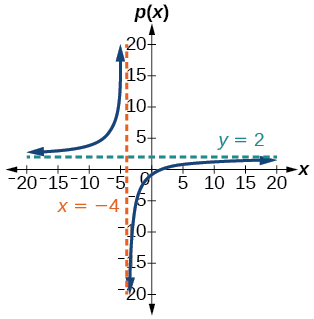40) $$q(x)=\dfrac{x-5}{3 x-1}$$

41) $$s(x)=\dfrac{4}{(x-2)^{2}}$$

V.A. $$x=2$$, H.A. $$y=0$$, $$(0,1)$$42) $$r(x)=\dfrac{5}{(x+1)^{2}}$$

43) $$f(x)=\dfrac{3 x^{2}-14 x-5}{3 x^{2}+8 x-16}$$

V.A. $$x=-4$$, $$x=\dfrac{4}{3}$$, H.A. $$y=1$$; $$(5,0)$$; $$\left(-\dfrac{1}{3}, 0\right)$$; $$\left(0, \dfrac{5}{16}\right)$$44) $$g(x)=\dfrac{2 x^{2}+7 x-15}{3 x^{2}-14+15}$$

45) $$a(x)=\dfrac{x^{2}+2 x-3}{x^{2}-1}$$

V.A. $$x=−1$$, H.A. $$y=1$$; $$(−3,0)$$; $$(0,3)$$46) $$b(x)=\dfrac{x^{2}-x-6}{x^{2}-4}$$

47) $$h(x)=\dfrac{2 x^{2}+x-1}{x-4}$$

V.A. $$x=4$$, S.A. $$y=2x+9$$; $$(-1,0)$$; $$\left(\dfrac{1}{2}, 0\right)$$; $$\left(0, \dfrac{1}{4}\right)$$48) $$k(x)=\dfrac{2 x^{2}-3 x-20}{x-5}$$

49) $$w(x)=\dfrac{(x-1)(x+3)(x-5)}{(x+2)^{2}(x-4)}$$

V.A. $$x=-2$$, $$x=4$$, H.A. $$y=1$$, $$(1,0)$$; $$(5,0)$$; $$(-3,0)$$; $$\left(0,-\dfrac{15}{16}\right)$$50) $$z(x)=\dfrac{(x+2)^{2}(x-5)}{(x-3)(x+1)(x+4)}$$

For the exercises 51-56, write an equation for a rational function with the given characteristics.

51) Vertical asymptotes at $$x=5$$, $$x$$ -intercepts at $$(2,0)$$ and $$(-1,0), y$$ -intercept at $$(0,4)$$

$$y=50 \dfrac{x^{2}-x-2}{x^{2}-25}$$

52) Vertical asymptotes at $$x=-4$$ and $$x=-1, x$$ -intercepts at $$(1,0)$$ and $$(5,0), y$$ -intercept at $$(0,7)$$

53) Vertical asymptotes at $$x=-4$$ and $$x=-5, x$$ -intercepts at $$(4,0)$$ and $$(-6,0),$$ Horizontal asymptote at $$y=7$$

$$y=7 \dfrac{x^{2}+2 x-24}{x^{2}+9 x+20}$$

54) Vertical asymptotes at $$x=-3$$ and $$x=6, x$$ -intercepts at $$(-2,0)$$ and $$(1,0),$$ Horizontal asymptote at $$y=-2$$

55) Vertical asymptote at $$x=-1,$$ Double zero at $$x=2, y$$ -intercept at $$(0,2)$$

$$y=\dfrac{1}{2} \dfrac{x^{2}-4 x+4}{x+1}$$

56) Vertical asymptote at $$x=3,$$ Double zero at $$x=1, y$$ -intercept at $$(0,4)$$

For the exercises 57-,64 use the graphs to write an equation for the function.

57)$$y=4 \dfrac{x-3}{x^{2}-x-12}$$

58)59)$$y=-9 \dfrac{x-2}{x^{2}-9}$$

60)61)$$y=\dfrac{1}{3} \dfrac{x^{2}+x-6}{x-1}$$

62)63)$$y=-6 \dfrac{(x-1)^{2}}{(x+3)(x-2)^{2}}$$

64)#### Numeric

For the exercises 65-69, make tables to show the behavior of the function near the vertical asymptote and reflecting the horizontal asymptote

65) $$f(x)=\dfrac{1}{x-2}$$

Vertical asymptote $$x=2$$, Horizontal asymptote \(